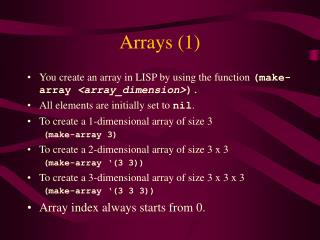DownloadDownload PresentationArrays (1)

# Arrays (1)

Download Presentation## Arrays (1)

- - - - - - - - - - - - - - - - - - - - - - - - - - - E N D - - - - - - - - - - - - - - - - - - - - - - - - - - -
##### Presentation Transcript

1. Arrays (1) • You create an array in LISP by using the function (make-array <array_dimension>). • All elements are initially set to nil. • To create a 1-dimensional array of size 3 (make-array 3) • To create a 2-dimensional array of size 3 x 3 (make-array ‘(3 3)) • To create a 3-dimensional array of size 3 x 3 x 3 (make-array ‘(3 3 3)) • Array index always starts from 0.

2. Arrays (2) • In the previous examples, after you have created the arrays, there was no way by which you could refer to it. • Here is how you can assign an array to a variable: (setq an_array (make-array 3)) • How would you then set and access the values of each cell within an array?

3. Aref… • To access a particular element in an array, use a function called aref. • For example, if you have a 1 dimensional array named an_array 10 elements, then to access the nth element, you could do this: (aref an_array n) For example: (aref an_array 9) (aref an_array 7)

4. Setf • Setf is the only way to set the elements of an array. • To set the nth element of an_array to a particular value, do as follows: (setf (aref an_array n) value) For example (setf (aref an_array 5) “Hello”)

5. Incf …(an interesting function?) • Incf reads a NUMBER from an array at a specified position, increments it, and then writes it back. • Assume an_array = #(“hello” NIL 3) • Then (incf (aref an_array 2)) will give you #(“hello” NIL 4) • What happens if you do this: (incf (aref an_array 0)) (incf (aref an_array 1))